# Animating thousands of fish with MultiMeshInstance¶

## Animating one Fish¶1. 从一边运动到另一边
2. 绕着鱼的中心作旋转运动
3. 平移波动运动
4. 平移扭转运动

```//time_scale is a uniform float
float time = TIME * time_scale;
```

```//side_to_side is a uniform float
VERTEX.x += cos(time) * side_to_side;
```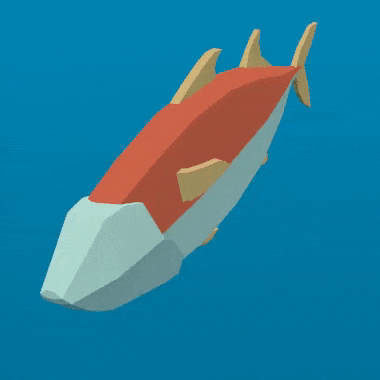```//angle is scaled by 0.1 so that the fish only pivots and doesn't rotate all the way around
//pivot is a uniform float
float pivot_angle = cos(time) * 0.1 * pivot;
mat2 rotation_matrix = mat2(vec2(cos(pivot_angle), -sin(pivot_angle)), vec2(sin(pivot_angle), cos(pivot_angle)));
```

```VERTEX.xz = rotation_matrix * VERTEX.xz;
```

With only the pivot applied you should see something like this: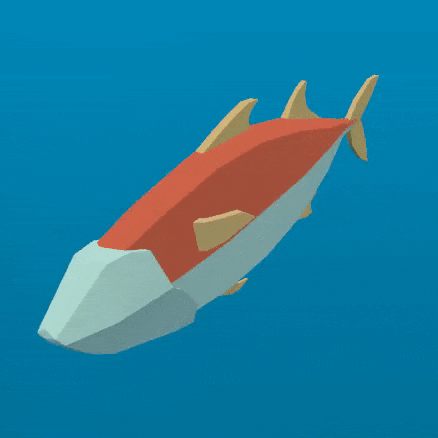```float body = (VERTEX.z + 1.0) / 2.0; //for a fish centered at (0, 0) with a length of 2
```

```//wave is a uniform float
VERTEX.x += cos(time + body) * wave;
```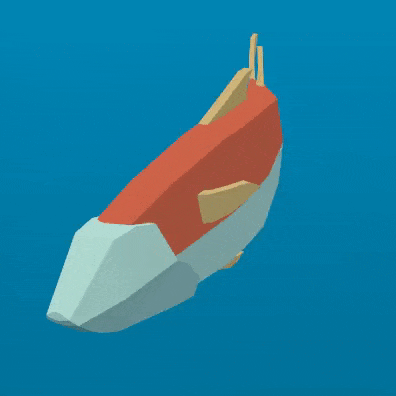```//twist is a uniform float
float twist_angle = cos(time + body) * 0.3 * twist;
mat2 twist_matrix = mat2(vec2(cos(twist_angle), -sin(twist_angle)), vec2(sin(twist_angle), cos(twist_angle)));
```

```VERTEX.xy = twist_matrix * VERTEX.xy;
```

Here is the fish with twist applied: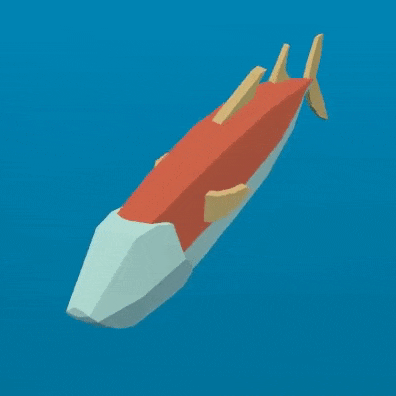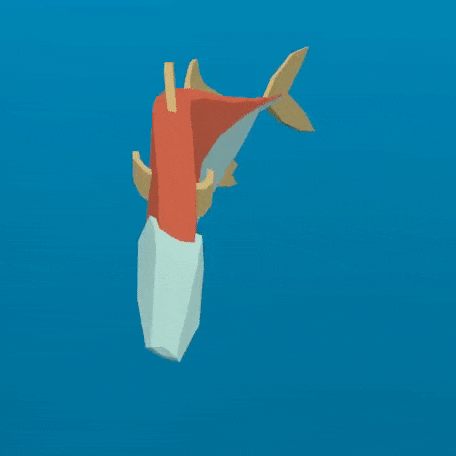“遮罩”是一个浮点数，它使用“平滑步进”控制从“0”到“1”之间发生转换的点，从鱼最前面的“0”到最后的“1”。

```//mask_black and mask_white are uniforms
``````//wave motion with mask
VERTEX.x += cos(time + body) * mask * wave;
```

```//twist motion with mask
VERTEX.xy = mix(VERTEX.xy, twist_matrix * VERTEX.xy, mask);
```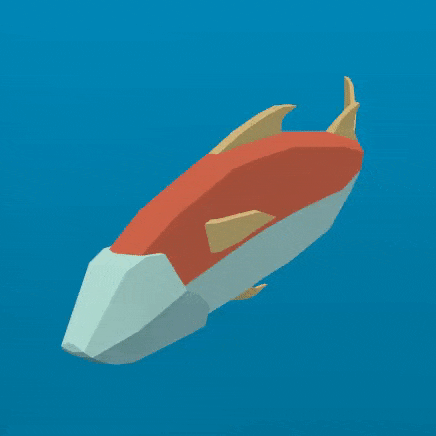## 制作一群鱼¶

Godot makes it easy to render thousands of the same object using a MultiMeshInstance node.

“实例_数量”指定要绘制的网格的实例数量。现在，将“实例_数量”保留为“0”，因为当“实例_数量”大于“0”时，您不能更改任何其他参数。我们稍后将在GDScript中设置“实例数量”。

“变换_格式”指定使用的变换是3D还是2D。对于本教程，选择3D。

```for i in range(\$School.multimesh.instance_count):
var position = Transform()
position = position.translated(Vector3(randf() * 100 - 50, randf() * 50 - 25, randf() * 50 - 25))
\$School.multimesh.set_instance_transform(i, position)
```

## Animating a school of fish¶

```float time = (TIME * time_scale) + (6.28318 * INSTANCE_CUSTOM.x);
```

```\$School.multimesh.set_instance_custom_data(i, Color(randf(), randf(), randf(), randf()))
```

```//set speed from 50% - 150% of regular speed
float time = (TIME * (0.5 + INSTANCE_CUSTOM.y) * time_scale) + (6.28318 * INSTANCE_CUSTOM.x);
```